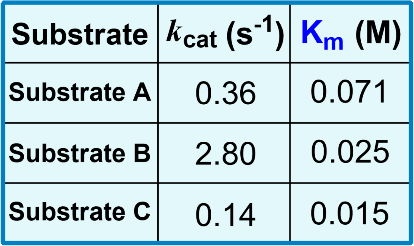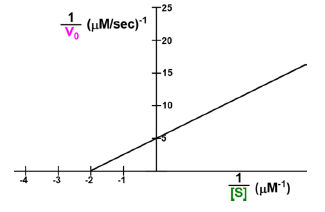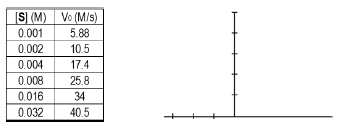Clutch Prep is now a part of Pearson
Ch. 6 - Enzymes and Enzyme KineticsWorksheetSee all chapters

# Specificity Constant

See all sections
Sections
Enzymes
Enzyme-Substrate Complex
Lock and Key Vs. Induced Fit Models
Optimal Enzyme Conditions
Activation Energy
Types of Enzymes
Cofactor
Catalysis
Electrostatic and Metal Ion Catalysis
Covalent Catalysis
Reaction Rate
Enzyme Kinetics
Rate Constants and Rate Law
Reaction Orders
Rate Constant Units
Initial Velocity
Vmax Enzyme
Km Enzyme
Michaelis-Menten Assumptions
Michaelis-Menten Equation
Lineweaver-Burk Plot
Michaelis-Menten vs. Lineweaver-Burk Plots
Shifting Lineweaver-Burk Plots
Calculating Vmax
Calculating Km
Kcat
Specificity Constant

Concept #1: Specificity Constant

###### Expert Q&A

Ask unlimited questions and get expert help right away.

Concept #2: Ratio of Kcat to Km Measures Catalytic Efficiency at Low [S]

###### Expert Q&A

Ask unlimited questions and get expert help right away.

Concept #3: Ratio of Kcat to Km Continued

###### Expert Q&A

Ask unlimited questions and get expert help right away.

Use the data in the chart below to provide answers to the following problems:

A) List the substrates from most preferred to least preferred under physiological conditions.

a) B, A, C.         b) C, B, A.         c) B, C, A.         d) A, C, B.B) List the substrates from most preferred to least preferred under saturating [S].

a) B, A, C.         b) C, B, A.         c) B, C, A.         d) A, C, B.

Concept #4: Diffusion-Controlled Limit of Specificity Constant

###### Expert Q&A

Ask unlimited questions and get expert help right away.

Practice: Which of the following options is correct concerning the turnover number (k cat) and the specificity constant?

Practice: Use the Lineweaver-Burk plot to help you calculate the Vmax, kcat, Km and specificity constant for the enzyme.

Assume the [E]T = 2.9 nM. Hint: Pay close attention to units.Vmax = ___________.

kcat = ___________.

Km = ___________.

kcat / Km = ___________.

Practice: Explain the steps you could take to accurately find the K m, Vmax, and specificity constant for an enzyme from the following kinetic data, assuming the experiments were all done with [E]T = 0.1 mM.Step #1: ___________________________________________

Step #2: ___________________________________________

Step #3: ___________________________________________

Step #4: ___________________________________________

Step #5: ___________________________________________

Practice: The specificity constant is obtained at low [S] via variable substitution into the Michaelis-Menten equation (Vmax = kcat[E]T). Considering this about the MM-equation, what is the relationship between changes in [S] & V0 when the [S] is super small and well below the Km?

###### Expert Q&A

Ask unlimited questions and get expert help right away.